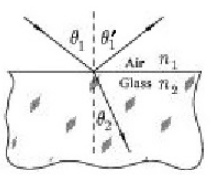# A light ray is incident on a reflecting surface. If the light ray makes a 25° angle

1. 1)A light ray is incident on a reflecting surface. If the light ray makes a 25° angle with respect to the normal to the surface, what is the exact angle (in degrees) made by the reflected ray relative to the normal?

2. 2)The light ray that makes the angle θ1’ is the refracted ray (true or false?)1. True or False: The Law of Reflection can be used to show how an image appears behind a mirror and it can also be used to determine where the image will be located.
1. Snell’s law is another name for the

2. A light ray incident on a block of glass makes an incident angle of 50.0° with the normal to the surface. The refracted ray in the block makes an 26.8° with the normal. What is the index of refraction of the glass?

1. A light ray traveling from a higher index of refraction medium into a lower index of refraction medium (for example, from water into air) would bend away from the normal to the medium surface. When the refracted ray is parallel to the boundary of the medium, θ2= 90°. The incident angle, θ1, becomes θc, called the